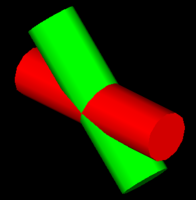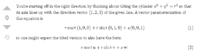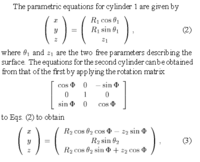# Intersection of 2 cylinders vector form

#### markraz

##### Junior Member
Hi am trying to understand the intersection of two cylinders that are not perpendicular. Either cylinder could be at any rotation in space.So would I need 3 unique vectors for U V W for each cylinder? total of 6 vectors?
would I set the equations up like this? where

v = is for vector
i, j, k are vector components for each vector
6 vectors = 1, 2, 3, 4, 5, 6
t, s, tt, ss = time

cylinder 1 vectors
u = v1
v = v2
w = v3
r1 cos(t) (v1i ,v1j , v1k) + r sin (t) (v2i ,v2j , v2k) + s (v3i, v3j, v3k)

cylinder 2 vectors
u = v4
v = v5
w = v6
r2 cos(tt) (v4i ,v4j , v4ak) + r sin (tt) (v5i ,v5j , v5k) + ss (v6i, v6j, v6k)

Is this how to set it up?
if so. once I setup these equations what do I do now to find the intersection? do I somehow solve for t and s?

#### Dr.Peterson

##### Elite Member
I don't know what your goal is, but it seems to me that the entire figure is determined by a vector for the axis of each cylinder, together with their radii. That's two vectors and two numbers. What three vectors would you be using for a cylinder??

#### markraz

##### Junior Member
I don't know what your goal is, but it seems to me that the entire figure is determined by a vector for the axis of each cylinder, together with their radii. That's two vectors and two numbers. What three vectors would you be using for a cylinder??
Hi Dr. Peterson TBH I don't even know if I need 3 vectors for each. I think I read UVW for each cylider in this articlei was referring to the cylinder equation
r (cost(t) u + sin(t) v + s * w
where u v and w are vectors??

thanks.

#### Dr.Peterson

##### Elite Member
Just read the rest of the answer to see what it says about u, v, and w, I guess.

But you should also consider whether a vector equation is what you need. I have no idea.

#### markraz

##### Junior Member
Just read the rest of the answer to see what it says about u, v, and w, I guess.

But you should also consider whether a vector equation is what you need. I have no idea.
Hi I was curious how cylinder/cylinder intersection worked. I don't know if the using vectors is the best way or could even be done. I was thinking using the vector equation would maybe make it easy to using matrices at some point maybe like this parametric form:

thanks#### Attachments

• 20.4 KB Views: 1

#### Cubist

##### Full Member
Your goal is to "understand the intersection of two cylinders that are not perpendicular". Can you explain further please? Let's assume that the two cylinders do actually intersect...

1) The description of the cylinders that you've posted are equations that define the surface of the cylinders (not the volume of the cylinders). Generally the intersection of two surfaces will result in one or more lines (unless some regions of the two surfaces coincide, and this exception would produce another surface). Such lines will be closed loops since both intersecting surfaces are "bound" (neither cylinder has holes in it)

2) Or are you thinking about the intersection of 2 cylindrical volumes? (the result of which will be another volume)

I assume that you're talking about scenario 1?

#### markraz

##### Junior Member
Your goal is to "understand the intersection of two cylinders that are not perpendicular". Can you explain further please? Let's assume that the two cylinders do actually intersect...

1) The description of the cylinders that you've posted are equations that define the surface of the cylinders (not the volume of the cylinders). Generally the intersection of two surfaces will result in one or more lines (unless some regions of the two surfaces coincide, and this exception would produce another surface). Such lines will be closed loops since both intersecting surfaces are "bound" (neither cylinder has holes in it)

2) Or are you thinking about the intersection of 2 cylindrical volumes? (the result of which will be another volume)

I assume that you're talking about scenario 1?
Hi thanks for the reply. TBH I am not even really sure how to explain it in math terminology. However this is the closest thing I could find:

So instead of surface/cylinder intersection I want to be able to find the vector equation of the cylinder/cylinder intersection.

This paper http://people.math.sfu.ca/~stockie/weld/weld.pdf is sort of what I am trying to do, however I am looking to find the vector equation for intersecting cylinders at any orientation in 3D space and not necessarily perpendicular.

I don't think am looking for volume since that would involve integration right?? I am just looking for a parametric or vector curve representation of the intersection result.

here is an other video:

I looked though all my books and there is no example of cylinder/cylinder intersection represented by vector equation.
Thanks any help would be appreciated

Last edited:

#### Cubist

##### Full Member
Sorry I made a mistake in my post by using the word "line" which implies a straight line. The proper word is "curve". Doh!

I don't think am looking for volume since that would involve integration right?? I am just looking for a parametric or vector curve representation of the intersection result.
I thought perhaps you may be studying 3d graphics, because your questions reminded me of the topic "constructive solid geometry" which is a way of ray-tracing shapes. Representing a volume involves the use of inequalities, for example the volume of a sphere would be x^2+y^2+z^2 ≤ r^2. But this isn't your goal.

Using equations similar to yours...

$$\displaystyle \boldsymbol{s_{v1}} = r_1\cos(t_1)\boldsymbol{v_1} + r_1\sin(t_1)\boldsymbol{v_2} + s_1\boldsymbol{v_3}$$

$$\displaystyle \boldsymbol{s_{v2}} = r_2\cos(t_2)\boldsymbol{v_4} + r_2\sin(t_2)\boldsymbol{v_5} + s_2\boldsymbol{v_6}$$

The first thing to do is equate them...

$$\displaystyle \boldsymbol{s_{v1}} =\boldsymbol{s_{v2}}$$

$$\displaystyle r_1\cos(t_1)\boldsymbol{v_1} + r_1\sin(t_1)\boldsymbol{v_2} + s_1\boldsymbol{v_3} = r_2\cos(t_2)\boldsymbol{v_4} + r_2\sin(t_2)\boldsymbol{v_5} + s_2\boldsymbol{v_6}$$

Next split out the x,y,z components and you'll end up with three equations. The unknowns are $$t_1, t_2, s_1, s_2$$. Using the three equations it should be possible to eliminate two of the unknowns and be left with an equation of the form:<something in terms of 2 unknowns> = 0. This "locks" the values of the two unknowns together onto a curve, and you can use these values to determine the eliminated unknowns by back substitution.

That's my suggestion for a "high level strategy", but the devil might be in the detail. I expect that a quadratic will show up somewhere, because it's possible to have two separated curves (imagine one cylinder has a smaller radius and it pierces the bigger cylinder in two separate locations). I guess you should aim to use the identity sin^2+cos^2=1. If you agree with this strategy then please give it a try and post your work.

I'm still a bit curious, are you doing this for interest? Do you have a computer controlled welder/ cutter?

#### markraz

##### Junior Member
Sorry I made a mistake in my post by using the word "line" which implies a straight line. The proper word is "curve". Doh!

I thought perhaps you may be studying 3d graphics, because your questions reminded me of the topic "constructive solid geometry" which is a way of ray-tracing shapes. Representing a volume involves the use of inequalities, for example the volume of a sphere would be x^2+y^2+z^2 ≤ r^2. But this isn't your goal.

Using equations similar to yours...

$$\displaystyle \boldsymbol{s_{v1}} = r_1\cos(t_1)\boldsymbol{v_1} + r_1\sin(t_1)\boldsymbol{v_2} + s_1\boldsymbol{v_3}$$

$$\displaystyle \boldsymbol{s_{v2}} = r_2\cos(t_2)\boldsymbol{v_4} + r_2\sin(t_2)\boldsymbol{v_5} + s_2\boldsymbol{v_6}$$

The first thing to do is equate them...

$$\displaystyle \boldsymbol{s_{v1}} =\boldsymbol{s_{v2}}$$

$$\displaystyle r_1\cos(t_1)\boldsymbol{v_1} + r_1\sin(t_1)\boldsymbol{v_2} + s_1\boldsymbol{v_3} = r_2\cos(t_2)\boldsymbol{v_4} + r_2\sin(t_2)\boldsymbol{v_5} + s_2\boldsymbol{v_6}$$

Next split out the x,y,z components and you'll end up with three equations. The unknowns are $$t_1, t_2, s_1, s_2$$. Using the three equations it should be possible to eliminate two of the unknowns and be left with an equation of the form:<something in terms of 2 unknowns> = 0. This "locks" the values of the two unknowns together onto a curve, and you can use these values to determine the eliminated unknowns by back substitution.

That's my suggestion for a "high level strategy", but the devil might be in the detail. I expect that a quadratic will show up somewhere, because it's possible to have two separated curves (imagine one cylinder has a smaller radius and it pierces the bigger cylinder in two separate locations). I guess you should aim to use the identity sin^2+cos^2=1. If you agree with this strategy then please give it a try and post your work.

I'm still a bit curious, are you doing this for interest? Do you have a computer controlled welder/ cutter?
Hi thanks for the detailed explanation. It's going to take me a while to figure this out ,but thanks so much. I'm sure I will have more questions.

But yeah I am doing 3D graphics. Please don't laugh (since it's obvious I am a novice) but I am writing a solid modeling CAD program for fun as a hobby. I have a good amount of it written over the last 7 years thanks to this site. You, Dr Peterson, Halls of Ivy, PKA and many others have really helped me quite a bit.

This particular intersection problem is something I am trying use to implement for "rounds" or fillets. Previously for 3d fillets I was just using an octtree and just intersecting polygon meshes ( more like the old autocad boolean way of doing it) . Like this:

I also used revolving surfaces or extruded arcs/circles and ellipses. It was sort of a sloppy implementation.

Recently someone I know who worked for PTC and Computervision back in the day, told me they used the vector functions for lots of the intersections with Breps. So I figured I would try it. (big mistake lol)

thanks again

Last edited:
•Cubist

#### Cubist

##### Full Member
I've done the calculation, and I got an answer that checks out. So I'll give you a few more high level clues now that I know a bit more...

I did it the following way:-
- consider adding a position offset to one(or both) of the cylinders, to make the intersection more flexible. I just added a v7 vector to sv2.
- start by eliminating s1 from the three equations (so you'll end up with 2 equations without s1)
- Then eliminate s2 to find one equation linking t1 and t2.

You don't need cos^2+sin^2=1. And no quadratics will show up. So much for my predictions!

The equation will be very long, but you can boil it down to this...

sin(t2)*cs2 + cos(t2)*cc2 = sin(t1)*cs1 + cos(t1)*cc1 + cns

where cs2,cc2,cs1,cc1, and cns are all scalar, constant, values and none of them contain any of:- trig, "t1" or "t2". They will contain many vector values and the radii

when you rearrange the above to t2=<something> form, then you obtain two possible values for t2 (and sometimes one, or zero values).

I'm very pleased that you're writing a CAD package as a hobby! It's a great way of improving your math AND coding skills. There's a good feeling of satisfaction when you run the code and you obtain a great looking image. I wrote a ray-tracing program years ago and had a lot of fun doing it (and a good measure of frustration sometimes too). I had primatives like sphere, cone, cylinder, disc etc. I eventually got "tired" of it and moved onto other hobby projects, but I might see if the old code still compiles this weekend (it was written in C++). I'm no longer up to speed with all the teminology that you're using! Nowadays I sometimes use the blender modelling package to visualise things, and I try to stick to shapes made up from nets of quadrilaterals because textures stretch very nicely over them, and they seem to produce great results when sub-divided for more detail (triangles aren't so nice).

#### markraz

##### Junior Member
I've done the calculation, and I got an answer that checks out. So I'll give you a few more high level clues now that I know a bit more...

I did it the following way:-
- consider adding a position offset to one(or both) of the cylinders, to make the intersection more flexible. I just added a v7 vector to sv2.
- start by eliminating s1 from the three equations (so you'll end up with 2 equations without s1)
- Then eliminate s2 to find one equation linking t1 and t2.

You don't need cos^2+sin^2=1. And no quadratics will show up. So much for my predictions!

The equation will be very long, but you can boil it down to this...

sin(t2)*cs2 + cos(t2)*cc2 = sin(t1)*cs1 + cos(t1)*cc1 + cns

where cs2,cc2,cs1,cc1, and cns are all scalar, constant, values and none of them contain any of:- trig, "t1" or "t2". They will contain many vector values and the radii

when you rearrange the above to t2=<something> form, then you obtain two possible values for t2 (and sometimes one, or zero values).
Thanks I'll try putting it all together now

I'm very pleased that you're writing a CAD package as a hobby! It's a great way of improving your math AND coding skills. There's a good feeling of satisfaction when you run the code and you obtain a great looking image. I wrote a ray-tracing program years ago and had a lot of fun doing it (and a good measure of frustration sometimes too). I had primatives like sphere, cone, cylinder, disc etc. I eventually got "tired" of it and moved onto other hobby projects, but I might see if the old code still compiles this weekend (it was written in C++). I'm no longer up to speed with all the teminology that you're using! Nowadays I sometimes use the blender modelling package to visualise things, and I try to stick to shapes made up from nets of quadrilaterals because textures stretch very nicely over them, and they seem to produce great results when sub-divided for more detail (triangles aren't so nice).
Yeah Raytracing is great. That was my first real interest in computers back in the 80s. It's amazing how far that technology has come with the PBR / BRDF and all the new lighting techniques. I suppose that stuff was around in the 80s but computers were so slow then you could even use those algorithms. I remeber it taking a couple days to render one image back then Now it can be done in real time with GPU.

Thanks again

#### Attachments

• 1.6 KB Views: 0
Last edited: